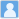• ### On the short-range behavior of neutrino forces beyond the Standard Model: from $1/r^5$ to $1/r^4$

分类： 天文学 >> 天文学 提交时间： 2023-02-19Xun-jie Xu Bingrong Yu

摘要：The exchange of a pair of neutrinos between two objects, seperated by a distance $r$, leads to a long-range effective potential proportional to $1/r_{}^5$, assuming massless neutrinos and four-fermion contact interactions. In this paper, we investigate how this known form of neutrino-mediated potentials might be altered if the distance $r$ is sufficiently short, corresponding to a sufficiently large momentum transfer which could invalidate the contact interactions. We consider two possible scenarios to open up the contact interactions by introducing a $t$-channel or an $s$-channel mediator. We derive a general formula that is valid to describe the potential in all regimes as long as the external particles remain non-relativistic. In both scenarios, the potential decreases as $1/r_{}^5$ in the long-range limit as expected. In the short-range limit, the $t$-channel potential exhibits the Coulomb-like behavior (i.e. proportional to $1/r$), while the $s$-channel potential exhibits $1/r_{}^4$ and $1/r_{}^2$ behaviors.

同行评议状态:待评议

• ### Invisible Neutrino Decays as Origin of TeV Gamma Rays from GRB221009A

分类： 天文学 >> 天文学 提交时间： 2023-02-19Jihong Huang Yilin Wang Bingrong Yu Shun Zhou

摘要：Recently, the LHAASO collaboration has observed the gamma rays of energies up to ten TeV from the gamma-ray burst GRB221009A, which has stimulated the community of astronomy, particle physics and astrophysics to propose various possible interpretations. In this paper, we put forward a viable scenario that neutrinos are produced together with TeV photons in the gamma-ray burst and gradually decay into the axion-like particles, which are then converted into gamma rays in the galactic magnetic fields. In such a scenario, the tension between previous axion-like particle interpretations and the existing observational constraints on the relevant coupling constant and mass can be relaxed.

同行评议状态:待评议

• ### Invisible Neutrino Decays as Origin of TeV Gamma Rays from GRB221009A

分类： 天文学 >> 天文学 提交时间： 2023-02-19Jihong Huang Yilin Wang Bingrong Yu Shun Zhou

摘要：Recently, the LHAASO collaboration has observed the gamma rays of energies up to ten TeV from the gamma-ray burst GRB221009A, which has stimulated the community of astronomy, particle physics and astrophysics to propose various possible interpretations. In this paper, we put forward a viable scenario that neutrinos are produced together with TeV photons in the gamma-ray burst and gradually decay into the axion-like particles, which are then converted into gamma rays in the galactic magnetic fields. In such a scenario, the tension between previous axion-like particle interpretations and the existing observational constraints on the relevant coupling constant and mass can be relaxed.

同行评议状态:待评议

• ### Neutrino forces in neutrino backgrounds

分类： 天文学 >> 天文学 提交时间： 2023-02-19Mitrajyoti Ghosh Yuval Grossman Walter Tangarife Xun-Jie Xu Bingrong Yu

摘要：The Standard Model predicts a long-range force, proportional to $G_F^2/r^5$, between fermions due to the exchange of a pair of neutrinos. This quantum force is feeble and has not been observed yet. In this paper, we compute this force in the presence of neutrino backgrounds, both for isotropic and directional background neutrinos. We find that for the case of directional background the force can have a $1/r$ dependence and it can be significantly enhanced compared to the vacuum case. In particular, background effects caused by reactor, solar, and supernova neutrinos enhance the force by many orders of magnitude. The enhancement, however, occurs only in the direction parallel to the direction of the background neutrinos. We discuss the experimental prospects of detecting the neutrino force in neutrino backgrounds and find that the effect is close to the available sensitivity of the current fifth force experiments. Yet, the angular spread of the neutrino flux and that of the test masses reduce the strength of this force. The results are encouraging and a detailed experimental study is called for to check if the effect can be probed.

同行评议状态:待评议